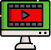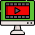VideosNotes

### Approximation

Quotient Rule.
In calculus, the quotient rule is a method of finding the derivative of a function that is the ratio of two differentiable functions.
Views: N/A
Video Duration: 4:47
Date Uploaded: November 18, 2020
Grade Level: SS 2
Share this with your friends!
On the Same TopicLOGARITHMS
Logarithm, the exponent or ...Logarithmic Equations
i. Revision of logarithm of...Anti-Logarithm
The anti-logarithm of a num...Logarithm - Quotient Rule
Since eln(x/y)=eln(x)−ln(y)...
Other Related Videos